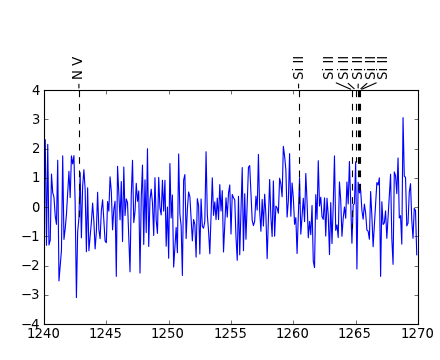# Line identification plots with automatic label layout¶

Manually placing labels in plots of spectrum is cumbersome. Functions in this module attempt to automatically position labels, in such a way that they do not overlap with each other.

The module can be installed using pip and easy_install:

`\$ pip install lineid_plot`

The source code is available at https://github.com/phn/lineid_plot .

The function plot_line_ids() takes several keyword parameters that can be used to customized the placement of labels. The labels are generated using the annotate function in Matplotlib. The function returns the Figure and Axes instances used, so that further customizations can be performed.

Some example are shown below.

## Minimal plot with automatic label layout¶

```import numpy as np
from matplotlib import pyplot as plt
import lineid_plot

wave = 1240 + np.arange(300) * 0.1
flux = np.random.normal(size=300)

line_wave = [1242.80, 1260.42, 1264.74, 1265.00, 1265.2, 1265.3, 1265.35]
line_label1 = ['N V', 'Si II', 'Si II', 'Si II', 'Si II', 'Si II', 'Si II']

lineid_plot.plot_line_ids(wave, flux, line_wave, line_label1)

plt.show()
```

[hires.png, pdf]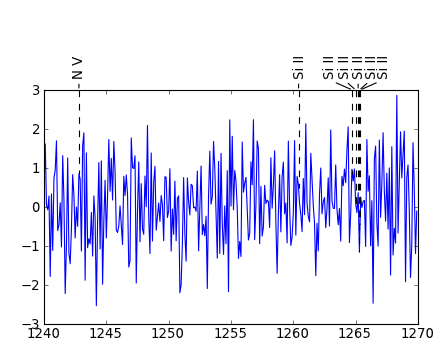## Plot without line from annotation point to flux level¶

```import numpy as np
from matplotlib import pyplot as plt
import lineid_plot

wave = 1240 + np.arange(300) * 0.1
flux = np.random.normal(size=300)

line_wave = [1242.80, 1260.42, 1264.74, 1265.00, 1265.2, 1265.3, 1265.35]
line_label1 = ['N V', 'Si II', 'Si II', 'Si II', 'Si II', 'Si II', 'Si II']

# Set extend=False.
lineid_plot.plot_line_ids(wave, flux, line_wave, line_label1, extend=False)

plt.show()
```

[hires.png, pdf]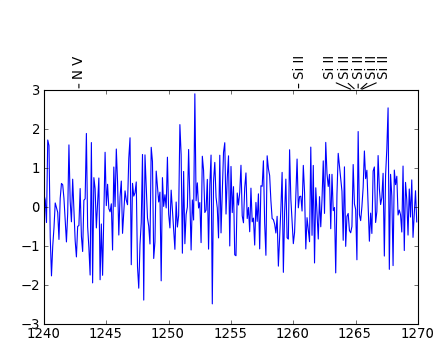## Multiple plots using user provided Axes instances¶

```import numpy as np
from matplotlib import pyplot as plt
import lineid_plot

wave = 1240 + np.arange(300) * 0.1
flux = np.random.normal(size=300)
line_wave = [1242.80, 1260.42, 1264.74, 1265.00, 1265.2, 1265.3, 1265.35]
line_flux = np.interp(line_wave, wave, flux)
line_label1 = ['N V', 'Si II', 'Si II', 'Si II', 'Si II', 'Si II', 'Si II']
label1_sizes = np.array([12, 12, 12, 12, 12, 12, 12])

fig = plt.figure()

# First Axes
ax.plot(wave, flux)

# Pass the Axes instance to the plot_line_ids function.
lineid_plot.plot_line_ids(wave, flux, line_wave, line_label1, ax=ax)

# Second Axes
ax1 = fig.add_axes([0.1, 0.55, 0.85, 0.35])
ax1.plot(wave, flux)

# Pass the Axes instance to the plot_line_ids function.
lineid_plot.plot_line_ids(wave, flux, line_wave, line_label1, ax=ax1)

plt.show()
```

[hires.png, pdf]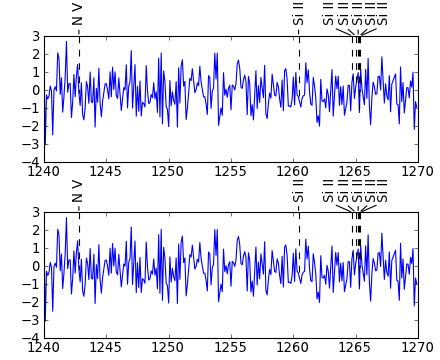## Applying small change to y axis location of label¶

By default the annotation point is placed on the y-axes upper bound and the box is placed 0.06 figure fraction units above the annotation point. Figure fraction units is used so that the appearance doesn’t depend on the y range of data. The default box location i.e., 0.06 can be changed using the keyword parameter box_axes_space.

It is better not to use large values for this keyword. Large values can lead to RuntimeError when Matplotlib tries to render the plot.

Examples of changing y axis locations of annotation points and label locations using data coordinates are show later. These methods are preferred when major changes are required.

```# box_axes_space takes numbers in figure fraction units. Keep this
# small.
lineid_plot.plot_line_ids(wave, flux, line_wave, line_label1,
box_axes_space=0.08)
```

[hires.png, pdf]## Custom y axis location for annotation points (arrow tips)¶

Use arrow_tip keyword to alter the annotation point. When assigning custom y axis locations, it is best to plot the data, set appropriate range for the Axes and then pass the Axes instance to plot_line_ids().

```# Use arrow_tip keyword.
lineid_plot.plot_line_ids(wave, flux, line_wave, line_label1,
arrow_tip=3.3, ax=ax)
```

[hires.png, pdf]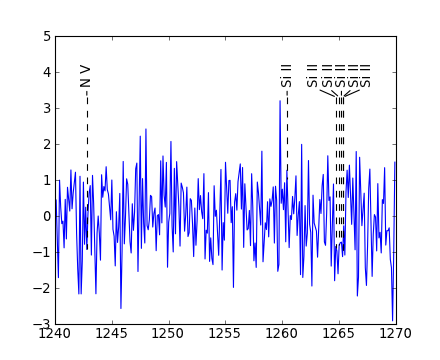Each label can have its own annotation point.

```arrow_tips = [3.3, 3.3, 3.3, 3.4, 3.5, 3.4, 3.3]
lineid_plot.plot_line_ids(wave, flux, line_wave, line_label1,
arrow_tip=arrow_tips, ax=ax)
```

[hires.png, pdf]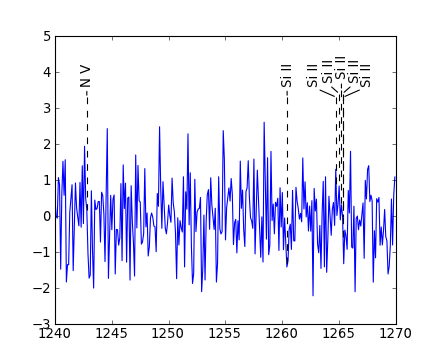## Custom y axis location for label boxes¶

The label boxes can be given a custom y axis location. The value given is taken as the y coordinate of the center of a box, in data coordinates. When assigning custom Y locations, it is best to plot the data, set appropriate range for the Axes and then pass the Axes instance to plot_line_ids().

```lineid_plot.plot_line_ids(wave, flux, line_wave, line_label1,
arrow_tip=3.3, ax=ax, box_loc=4.3)
```

[hires.png, pdf]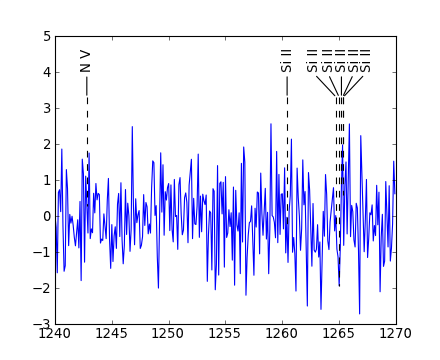Each box can be assigned a separate Y box location.

```arrow_tips = [3.3, 3.3, 3.3, 3.4, 3.5, 3.4, 3.3]
box_loc = [4.3, 4.3, 4.3, 4.4, 4.5, 4.4, 4.3]
lineid_plot.plot_line_ids(wave, flux, line_wave, line_label1,
arrow_tip=arrow_tips, box_loc=box_loc, ax=ax)
```

[hires.png, pdf]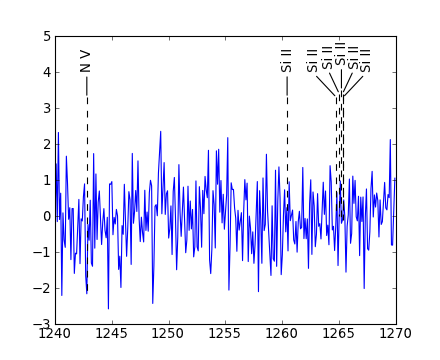## Accessing a specific label¶

Each box has a property named label. These are identical to the input labels, except when there are duplicated. The duplicate texts are given a numeric suffix, based on the order in which the duplicates occur in the input. These are generated using the unique_labels function.

```>>> line_label1
['N V', 'Si II', 'Si II', 'Si II', 'Si II', 'Si II', 'Si II']
>>> lineid_plot.unique_labels(line_label1)
['N V',
'Si II_num_1',
'Si II_num_2',
'Si II_num_3',
'Si II_num_4',
'Si II_num_5',
'Si II_num_6']
```

Each line extending from the annotation point to the flux level, is also assigned a label properties. The value is the above label property suffixed with “_line”.

Matplotlib Figure and Axes instances have a method findobj() which can be used to find objects in it, that satisfy certain conditions. For example, all Annotation objects. It will accept a function, and any object that will cause this function to return True, will be returned by findobj().

In the following one of the “Si II” boxes and line extending to the flux level are made invisible.

```import numpy as np
from matplotlib import pyplot as plt
import matplotlib as mpl
import lineid_plot

wave = 1240 + np.arange(300) * 0.1
flux = np.random.normal(size=300)
line_wave = [1242.80, 1260.42, 1264.74, 1265.00, 1265.2, 1265.3, 1265.35]
line_flux = np.interp(line_wave, wave, flux)
line_label1 = ['N V', 'Si II', 'Si II', 'Si II', 'Si II', 'Si II', 'Si II']
label1_sizes = np.array([12, 12, 12, 12, 12, 12, 12])

fig = plt.figure()
ax.plot(wave, flux)
ax.axis([1240, 1270, -3, 5])

arrow_tips = [3.3, 3.3, 3.3, 3.4, 3.5, 3.4, 3.3]
box_loc = [4.3, 4.3, 4.3, 4.4, 4.5, 4.4, 4.3]
lineid_plot.plot_line_ids(wave, flux, line_wave, line_label1,
arrow_tip=arrow_tips, box_loc=box_loc, ax=ax)

a = ax.findobj(mpl.text.Annotation)
for i in a:
if i.get_label() == "Si II_num_4":
i.set_visible(False)

a = ax.findobj(mpl.lines.Line2D)
for i in a:
if i.get_label() == "Si II_num_4_line":
i.set_visible(False)

plt.show()
```

[hires.png, pdf]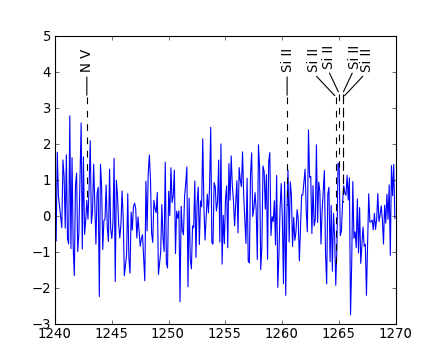## Customizing box location adjustment parameters¶

The parameter max_iter fixes the maximum number of adjustments to perform. Parameter adjust_factor sets the factor by which the current separation of the boxes must be increased or decreased. After fd_p percentage of max_iter the adjust_factor is reduced by a factor of factor_decrement. The defaults for all these should be enough in most situations.

The example below shows, for the data used, a low value of max_iter doesn’t separate the labels well enough.

```lineid_plot.plot_line_ids(wave, flux, line_wave, line_label1,
max_iter=10)
```

[hires.png, pdf]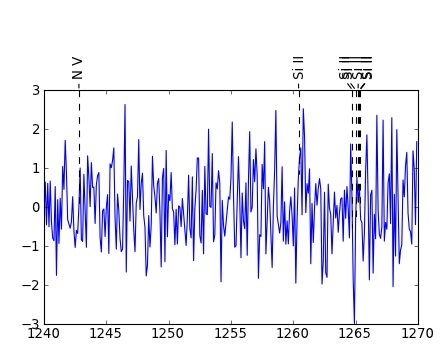Using a value of 300 works. Note that the default is 1000.

```lineid_plot.plot_line_ids(wave, flux, line_wave, line_label1,
max_iter=300)
```

[hires.png, pdf]